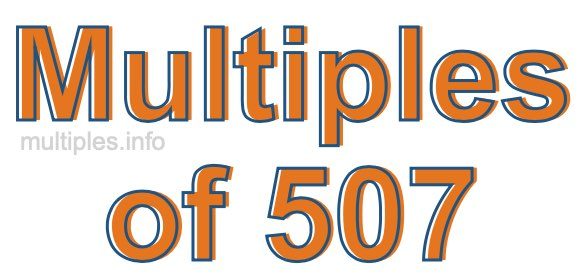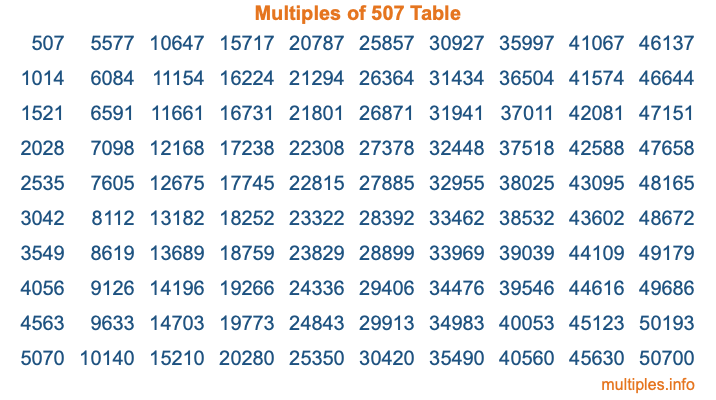Multiples of 507Welcome to the Multiples of 507 page. Here we will first teach you everything you will ever need to know about the multiples of 507, and then give you a study guide summary of everything we taught you to make sure you remember it all. Use this page to look up facts and learn information about the multiples of 507. This page will make you a multiples of five hundred seven expert!

Definition of Multiples of 507
Multiples of 507 are all the numbers that when divided by 507 equal an integer. Each of the multiples of 507 are called a multiple. A multiple of 507 is created by multiplying 507 by an integer.

Therefore, to create a list of multiples of 507, you start with 1 multiplied by 507, then 2 multiplied by 507, then 3 multiplied by 507, and so on for as long as you want. Thus, the list of the first five multiples of 507 is 507, 1014, 1521, 2028, and 2535. To see a larger list of multiples of 507, see the printable image of Multiples of 507 further down on this page. We also have a category where you can choose any nth multiple of 507.

Multiples of 507 Checker
The Multiples of 507 Checker below checks to see if any number of your choice is a multiple of 507. In other words, it checks to see if there is any number (integer) that when multiplied by 507 will equal your number. To do that, we divide your number by 507. If the the quotient is an integer, then your number is a multiple of 507.

Is  a multiple of 507?

Least Common Multiple of 507 and ...
A Least Common Multiple (LCM) is the lowest multiple that two or more numbers have in common. This is also called the smallest common multiple or lowest common multiple and is useful to know when you are adding our subtracting fractions. Enter one or more numbers below (507 is already entered) to find the LCM.

Check out our LCM Calculator if you need more details about the Least Common Multiple or if you need the LCM for different numbers for adding and subtraction fractions.

nth Multiple of 507
As we stated above, 507 is the first multiple of 507, 1014 is the second multiple of 507, 1521 is the third multiple of 507, and so on. Enter a number below to find the nth multiple of 507.

th multiple of 507

Multiples of 507 vs Factors of 507
507 is a multiple of 507 and a factor of 507, but that is where the similarities end. All postive multiples of 507 are 507 or greater than 507. All positive factors of 507 are 507 or less than 507.

Below is the beginning list of multiples of 507 and the factors of 507 so you can compare:

Multiples of 507: 507, 1014, 1521, 2028, 2535, etc.

Factors of 507: 1, 3, 13, 39, 169, 507

As you can see, the multiples of 507 are all the numbers that you can divide by 507 to get a whole number. The factors of 507, on the other hand, are all the whole numbers that you can multiply by another whole number to get 507.

It's also interesting to note that if a number (x) is a factor of 507, then 507 will also be a multiple of that number (x).

Multiples of 507 vs Divisors of 507
The divisors of 507 are all the integers that 507 can be divided by evenly. Below is a list of the divisors of 507.

Divisors of 507: 1, 3, 13, 39, 169, 507

The interesting thing to note here is that if you take any multiple of 507 and divide it by a divisor of 507, you will see that the quotient is an integer.

Multiples of 507 Table
Below is an image of the first 100 multiples of 507 in a table. The table is in chronological order, column by column. The first column has the first ten multiples of 507, the second column has the next ten multiples of 507, and so on.The Multiples of 507 Table is also referred to as the 507 Times Table or Times Table of 507. You are welcome to print out our table for your studies.

Negative Multiples of 507
Although not often discussed or needed in math, it is worth mentioning that you can make a list of negative multiples of 507 by multiplying 507 by -1, then by -2, then by -3, and so on, to get the following list of negative multiples of 507:

-507, -1014, -1521, -2028, -2535, etc.

Multiples of 507 Summary
Below is a summary of important Multiples of 507 facts that we have discussed on this page. To retain the knowledge on this page, we recommend that you read through the summary and explain to yourself or a study partner why they hold true.

There are an infinite number of multiples of 507.

A multiple of 507 divided by 507 will equal a whole number.

507 divided by a factor of 507 equals a divisor of 507.

The nth multiple of 507 is n times 507.

The largest factor of 507 is equal to the first positive multiple of 507.

507 is a multiple of every factor of 507.

507 is a multiple of 507.

A multiple of 507 divided by a divisor of 507 equals an integer.

507 divided by a divisor of 507 equals a factor of 507.

Any integer times 507 will equal a multiple of 507.

Multiples of a Number
Here you can get the multiples of another number, all with the same attention to detail as we did for multiples of 507 on this page.

Multiples of
Multiples of 508
Did you find our page about multiples of five hundred seven educational? Do you want more knowledge? Check out the multiples of the next number on our list!